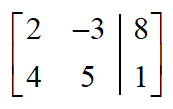index: click on a letter A B C D E F G H I J K L M N O P Q R S T U V W X Y Z A to Z index index: subject areas numbers & symbols sets, logic, proofs geometry algebra trigonometry advanced algebra & pre-calculus calculus advanced topics probability & statistics real world applications multimedia entrieswww.mathwords.com about mathwords website feedback

Augmented Matrix

A matrix form of a linear system of equations obtained from the coefficient matrix as shown below. It is created by adding an additional column for the constants on the right of the equal signs. The new column is set apart by a vertical line.

 System: $$\begin{array}{l}2x - 3y = 8\\4x + 5y = 1\end{array}$$ Augmented Matrix: